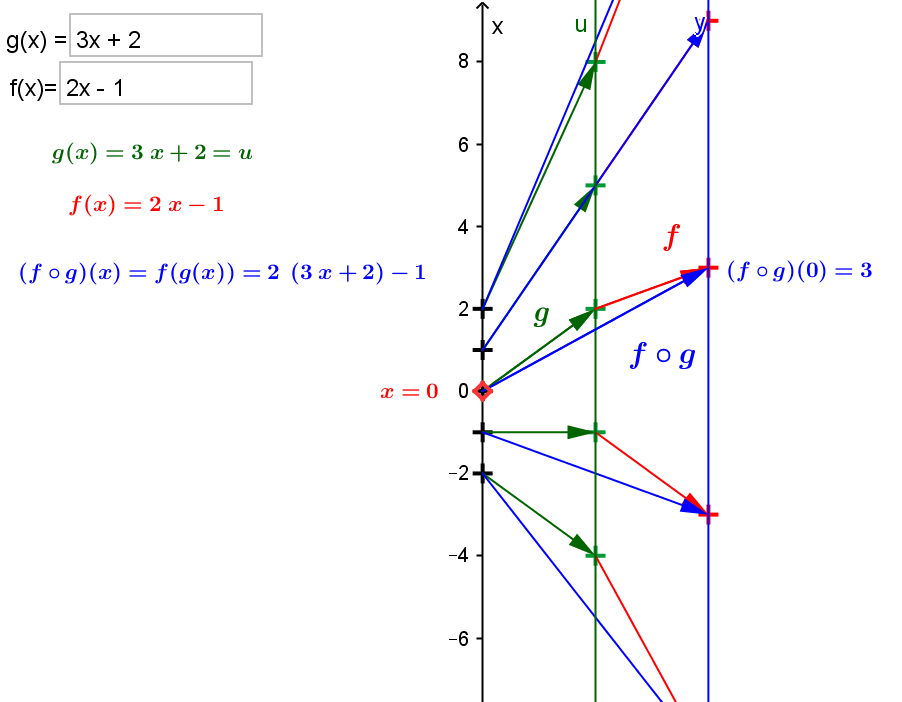Example  1.   \$ f(x) = 2x - 1; g(x) = 3x + 2\$.

\$(f \circ g)(x) = f(g(x)) =f(3x+2) = 2*(3x+2) - 1 = (6x +4) -1= 6x + 3\$Select the point \$x\$ in the mapping diagram to move the point by mouse or use the scroll up or down key  to move by increments.
The slider \$n\$ determines the number of points in the domain for the mapping diagram.